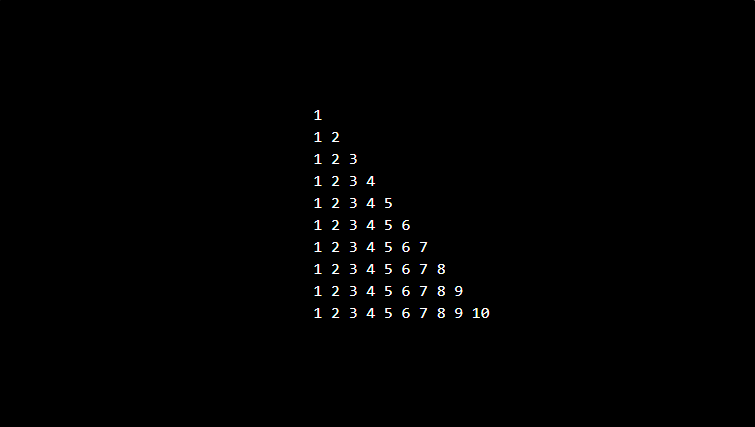In this article write a PHP program to print triangle number. We run for loop 10 times, in which you have 1 outer for loops which contains 1 nested for loop and if condition to print triangle number as shown in the following program.

## Program to print Triangle number

```<?php
echo "<pre>";
for(\$i=1;\$i<=10;\$i++){
for(\$j=1;\$j<=\$i;\$j++){
echo \$j."&nbsp;";
if(\$j==\$i){
echo "";
echo "<br/>";
}
}
}
echo "</pre>";
?>
```

### Output: